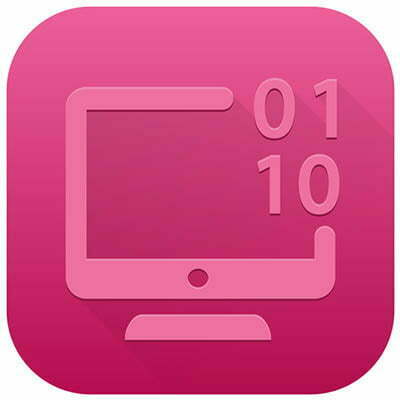# Tech Term: Bits and Bytes

Running a business sometimes requires attention to very minute details, and some things must be measured in order to achieve optimal efficiency. You’ve likely heard the terms bits and bytes used regarding data storage or transfer, but do you know what the difference is between them? Today’s tech term is dedicated to this explanation.

One of the first things you’ll notice when you go to buy a new computer is how much data the hard drive can store and how much random access memory (RAM) it contains. You might see numbers like 500GB or 2TB. The easiest way to explain this is by looking at the basics of data measurement. You can think of a bit as the smallest form of data measurement on a computer. Computers use binary math to showcase each potential digit as a bit. Each bit has a value of a 0 or 1. These bits are generated by the computer’s electrical current that activates the various internal components. These changes in voltage are used to transmit the bits, process calculations, and relay data across the network.

Here are some of the methods used during network message encoding:

• Wi-Fi carries bits using radio signals
• Ethernet connections carry bits using electric signals of varying voltages
• Fiber connections use pulses of light to carry bits

Ideally, the bits are encrypted so that they can’t be interpreted without permission.

On the other hand, the byte is a fixed sequence of bits. Technology today relies on organizing data into bytes to increase the speed and efficiency of data processing. Bits are often too small to measure data, which is why a byte is easier to use as the standard measurement.

The rate at which a computer network connection is measured is through time (bits per second), and today’s technology has advanced so far that it can transmit millions, or even billions, of bits per second (called megabits (Mbps) or gigabits (Gbps). The speed at which this data is transferred depends on the size of the file sizes or components transferring the data.

This is one of the reasons why gigabit network switches and other devices exist. If a device can support 1 Gbps, it transfers a single gigabit per second. Depending on your infrastructure, you might need to transfer more than this amount of data so that the network can operate smoothly. Other devices on your network will also play a major role in determining what your overall maximum speed is.

Breaking Down the Numbers
Since every byte is eight bits, you could safely assume that a kilobyte is 1,000 bytes, but you would be mistaken. Computers use binary systems, so your hard drives, memory, and bandwidth are all measured in powers of two. Thus, 2 ^ 10 equals 1,024, not 1,000. This makes looking at the specific numbers somewhat confusing for the average user.

If you look at everyday examples of this in practice, it becomes a little easier to understand and work with. Take a look at your IP address. This contains a string of 32 bits (or four bytes). An IP address with a value of 192.168.1.1 has values of 192, 168, 1, and 1 for each of its bytes. If you look at the encoding of this IP address, it would look like this:

11000000 10101000 00000001 000000001

This means that:

• 192 = 1100000
• 168 = 1010100
• 1 = 00000001

How to Convert Bits to Bytes (and Beyond)
If you ever need to convert bits to bytes or otherwise, here are the numbers.

• 8 bits = 1 byte
• 1,024 bytes = kilobyte
• 1,024 kilobytes = megabyte
• 1,024 megabytes = gigabyte
• 1,024 gigabytes = terabyte

If you want to convert four kilobytes into bits, you need to first convert the kilobytes to bytes (4 x 1,024) and then use that total (4,096) to convert to bits (8 x 4,096 = 32,768).

From a consumer standpoint, if you purchase a hard drive that has a terabyte of data, it’s real value is about 8 trillion bits. Hard drive manufacturers measure content by rounding down to 1,000 megabytes per gigabyte, even though most computers will use the 1,024 number. This is why when you purchase a new terabyte hard drive, you’ll notice that about 35 gigabytes aren’t immediately available. In the case of a workstation, the operating system will also consume a certain amount of data on the drive.

Did we answer some of your questions about computing and the specifics of bits vs bytes? Let us know in the comments what you would like to see covered in our tech term articles.